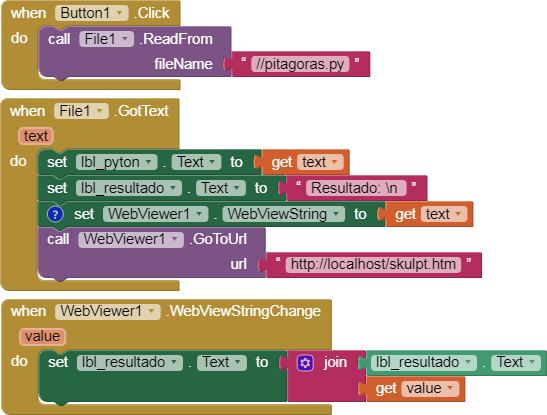# Testing Python in App Inventor with Skulpt

In this tutorial @Taifun develops a tutorial to run Python code using Skulpt. Thank you @Taifun!

We're going to try it.

I'm going to simplify the Skulpt code and the block code a bit, here they are.

skulpt.htm

``````<script src="jquery-1.9.0.min.js"></script>
<script src="skulpt.min.js"></script>
<script src="skulpt-stdlib.js"></script>
<script>
if (Sk.builtinFiles === undefined || Sk.builtinFiles["files"][x] === undefined)
return Sk.builtinFiles["files"][x];
}

function outf(text) {
window.AppInventor.setWebViewString("" + text);
}

var prog = window.AppInventor.getWebViewString();
try {
Sk.importMainWithBody("<stdin>",false,prog);
} catch (e) {
}
</script>
``````

pitagoras.py

``````import math
cateto1 = input("Valor de un cateto: ")
cateto2 = input("Valor del otro cateto: ")
hipotenusa = math.sqrt(math.pow(int(cateto1),2) + math.pow(int(cateto2),2))
print(hipotenusa)
``````p170A_pythonpitagoras.aia (151.0 KB)

There are still lots of things to implement under the hood, but we have made a huge leap forward in Python 3 compatibility. We will still support Python 2 as an option going forward for projects that rely on it. from https://skulpt.org/

You can try the Python code at:

9 Likes

Thank you @Juan_Antonio for the update...

Taifun

3 Likes

Thank you for this @Juan_Antonio and @Taifun

Is it possible to IMPORT eg, a variable or other value, from AI2 into Skulpt/Python, process it there, and then output the result back into AI2 as you show here?

Could you kindly explain the pros and cons of using AI2 blocks vs an extension vs Skulpt/Python. Thanks.

pitagoras2.py

``````import math
hipotenusa = math.sqrt(math.pow(int(cateto1),2) + math.pow(int(cateto2),2))
print(hipotenusa)
``````

p170A_pythonpitagoras_v3.aia (151.5 KB)

• In Python the correct placement of spaces and tabs is very important, a misplaced space will cause an error.

• Extensions are used when we need an algorithm that cannot be done with blocks or when we need many blocks to do it.

Sometimes old extensions cause problems when new versions of Android come out.

We can also use JavaScript, through JS we can perform mathematical operations much faster than with blocks.

Skulpt is a system that compiles Python (of the 2.6-ish variety) into Javascript, it's a limited library.

Thanks for the fast and clear reply @Juan_Antonio. Much appreciated.

Will experiment some more...

This .py has the same calculation duplicated as result1 & 2 but includes int/float on the second version. Variable n6 is not used in these particular calculations.

CodeSculpt gives the same correct result (198) for both versions:

However, when used in AI2 the Results1 & 2 are 218 and 198 respectively.

CodeSculpt_PythonFloat.aia (151.0 KB)

In AI2 is it therefore essential to use the int/float within any calculations or have I missed a trick?

The problem isn't AI2, it's the way Skulpt adapts the code to Python.
It can be fixed by declaring the variable type.
n1 = float(118)
n2 = float(1)
n3 = float(31)
n4 = float(90)
n5 = float(41)
n6 = float(0)

1 Like

Once again, many thanks @Juan_Antonio

1 Like

Hi, it possible run upload image in Python ? Multipart/form-data

for import requests show error `Line 1: ImportError: No module named requests`

Summary
``````import requests
import json

token ='12345'
group_id ='12345'